Courses

# Surveying (Part - 1) Civil Engineering (CE) Notes | EduRev

## Civil Engineering (CE): Surveying (Part - 1) Civil Engineering (CE) Notes | EduRev

The document Surveying (Part - 1) Civil Engineering (CE) Notes | EduRev is a part of the Civil Engineering (CE) Course Civil Engineering SSC JE (Technical).
All you need of Civil Engineering (CE) at this link: Civil Engineering (CE)

Chapter 1 Introduction
SURVEYING
Surveying is the process of determining relative positions of different objects on the surface of the earth by measuring horizontal distances between them and preparing a map to any suitable scale. Measurements are taken in a horizontal plane.

Reconnaissance:

•This is an exhaustive preliminary survey of the land to be surveyed. It may be either ground reconnaissance or aerial reconnaissance survey.
• Reconnaissance is made on arrival to site during which an overall picture or view of the area is obtained. The most suitable position of stations is selected, the purpose of the survey and the accuracy required will be drawn, and finally, the method of observation will be established.

Objectives of reconnaissance:
1. To ascertain the possibility of building or constructing route or track through the area.
2. To choose the best one or more routes and record on a map
3. To estimate probable cost and draft a report.

Principle of working from whole to part:
-It is a fundamental rule to always work from the whole to the part. This implies a      precise control surveying as the first consideration followed by subsidiary detail     surveying.

-This surveying principle involves laying down an overall system of stations whose positions are fixed to a fairly high degree of accuracy as control, and then the survey of details between the control points may be added on the frame by less elaborate methods.
-Once the overall size has been determined, the smaller areas can be surveyed in the knowledge that they must (and will if care is taken) put into the confines of the main overall frame.
-Errors which may inevitably arise are then contained within the framework of the control points and can be adjusted to it.

The surveying may primarily be divided into two divisions :
1. Plane surveying
2. Geodetic surveying
1. Plane surveying : The surveys in which earth surface is assumed as a plane and the curvature of the earth is ignored, are known as plane surveys.

2. Geodetic surveying : The surveys in which curvature of the earth is taken into account and higher degree of accuracy in linear as well as angular observations is achieved, are known as Geodetic surveying.

Classification based on Instrument used :
(1) Chain surveying
(2) Compass surveying
(3) Plane table surveying
(4) Theodolite surveying
(5) Tacheometric surveying
(6) Triangulation surveying
(7) Aerial surveying
(8) Photogrammetric surveying

MAP : The representation of the earth surface on a small scale, is called a map.

Units of Measurement, : There are two kinds of measurements used in plane surveying:
1. Linear measurement i.e. horizontal or vertical distances.
2. Angular measure i.e. horizontal or vertical angles.
-Scales on the maps are represented by the following two methods:
(i) Numerical scales
(ii) Graphical scales
(a) Engineer’s scale
(b) Fractional scale

(a) Engineer’s scale : The scale on which one cm on the plan represents some whole number of metres in the ground, is known as Engineer’s scale, e.g., 1 cm = 5 m, 1 cm = 10 m etc.

(b) Fractional scale : The scale on which an unit of length on the plan represents some number of the same unit of length on the ground is known as frictional scale, e.g , 1:500. 1:1000, 1:5000 etc.
Scales

Scale is the fixed ratio that every distance on the plan bears with corresponding distance on the ground.

Representative Fraction (RF)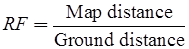Classification of Scales : The scales drawn on the maps or plans, may be classified as:
(i) Plain scale
(ii) Diagonal scale
(iii) Scale of chords
(iv) Vernier scale
-Plain Scale : A plane scale is one on which it is possible to measure only two dimensions, i.e. metres and decimetre, kilometres and hectametres, miles and furlongs etc.

Plain scales as Recommended by IS 1481-1959
Full size – 1 : 1
50 cm to a metre – 1 : 2
40 cm to a metre – 1 : 2.5
20 cm to a metre – 1 : 5
10 cm to a metre – 1 : 10 
-A useful map scale should be sufficiently long and should not be less than 19 cm and more than 32 cm.

(i) Diagonal scales : On a diagonal scale, it is possible to measure three dimensions such as kilometres, hectametres and decametres; or yards, feet and inches etc.

(ii) Vernier scales : It consists of two approximating scales, one of them is fixed and is called the primary scale, the other is movable and is called the vernier.

Least count of a vernier is equal to the difference between the smallest division on the vernier scale.
-Least count (L-C) =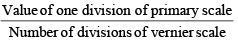-1 hectares = 10, 000 m2
-If 1 cm = 50 m, then R.F.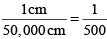Error Due to Use of the Wrong Scale

1. Correct Length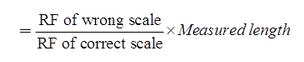2. Correct Length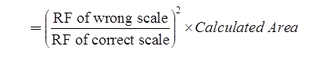Error Due to Incorrect Length of Chain or Type

(a) True length of the line, (l)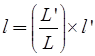Here, L = Designated length of tape/chain
L’ = Actual but wrong length of the chain or tape
l’ = Wrong measured length of the line
l = Actual true length of the line

Case (a): In case of Area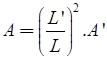where, A = True area
A’ = Wrong measured area

Case (b): In case of volume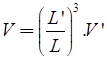where, V = True volume
V' = Wrong measured volume

The document Surveying (Part - 1) Civil Engineering (CE) Notes | EduRev is a part of the Civil Engineering (CE) Course Civil Engineering SSC JE (Technical).
All you need of Civil Engineering (CE) at this link: Civil Engineering (CE)Use Code STAYHOME200 and get INR 200 additional OFF Use Coupon Code
All Tests, Videos & Notes of Civil Engineering (CE): Civil Engineering (CE)

### Top Courses for Civil Engineering (CE)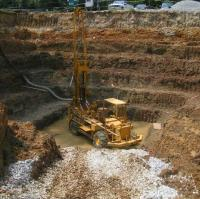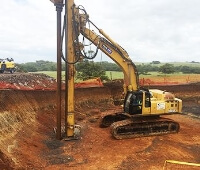## Civil Engineering SSC JE (Technical)

109 docs|50 tests

### Top Courses for Civil Engineering (CE)Track your progress, build streaks, highlight & save important lessons and more!

,

,

,

,

,

,

,

,

,

,

,

,

,

,

,

,

,

,

,

,

,

;